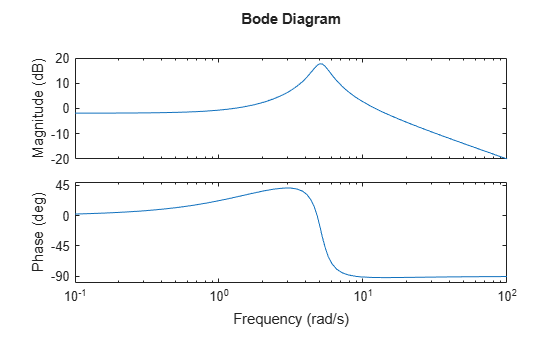# Frequency Response of a SISO System

This example shows how to plot the frequency response and obtain frequency response data for a single-input, single-output (SISO) dynamic system model.

Create a transfer function model and plot its frequency response.

```H = tf([10,21],[1,1.4,26]); bode(H)```When you can `bode` without output arguments, it plots the frequency response on the screen. Unless you specify a frequency range to plot, `bode` automatically chooses a frequency range based on the system dynamics.

Calculate the frequency response between 1 and 13 rad/s.

`[mag,phase,w] = bode(H,{1,13});`

When you call `bode` with output arguments, the command returns vectors `mag` and `phase` containing the magnitude and phase of the frequency response. The cell array input `{1,13}` tells `bode` to calculate the response at a grid of frequencies between 1 and 13 rad/s. `bode` returns the frequency points in the vector `w`.